Sec. 2.9 described the conservation and boundedness qualities of the advective derivatives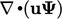and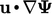, respectively. According to Eq. (2.31 ), the terms are equivalent when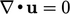, i.e. when mass conservation Eq. (2.8 ) is satisﬁed in the case when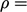constant.

During computation of an incompressible ﬂow, numerical error can be signiﬁcant, in particularly at the beginning of a steady-state calculation. The governing equations are not satisﬁed, including mass conservation, so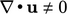.

Discretisation of an advection term of the form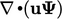as described in Sec. 3.9 can cause unboundedness in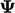. Ifhas a physical bound that is violated, the solution rapidly becomes unstable.

The addition of the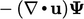term ensures boundedness while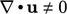, at the expense of conservation. Once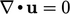, the solution is both bounded and conservative.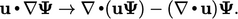(3.43)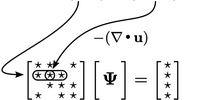This additional term is implicit in, so contributes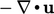to the diagonal coeﬃcients of the matrix. If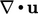is positive, we might expect a singular matrix, as discussed in Sec. 3.20 . However, the decrease to the diagonal coeﬃcient is matched by an increase from the discretisation of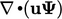. If that term uses the upwind scheme, the discretisation can be split into a contribution from positive outgoing ﬂuxes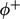and negative incoming ﬂuxes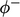. In extensive form (i.e. scaled by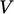, see Sec. 3.24 ), using values in the cell of interest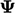and neighbouring cells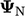, the terms are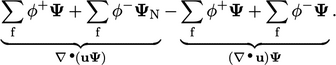The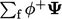terms cancel, leaving negative coeﬃcients for neighbouring cells (since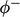is negative). The diagonal coeﬃcient is then equal to the sum of magnitude of those neighbour cell coeﬃcients, resulting in an invertible matrix.

### Capturing physics

Boundedness and conservation can be compromised when a term in an equation does not capture the physics of the problem. An example from ﬂame speed combustion modelling uses a parameterwhich represents the fraction of the unburnt fuel mixture.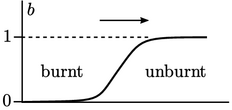The equation forincludes the source term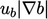, where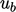is a calculated ﬂame speed. Its inclusion could cause the solution ofto fall below its lower bound of 0.

Multiplying and dividing by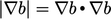changes the term to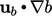, where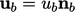and unit vector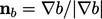. In this form, the term represents the non-conservative advection ofby a ﬂame velocity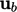moving in the direction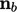, which captures the physical nature of combustion. Boundedness can then be maintained by a suitable choice of advection scheme.

Notes on CFD: General Principles - 3.22 Bounded advection discretisation